# Transformer 结构介绍2022年10月24日07:58:14

6683字阅读22分16秒

## 简介

`Seq2Seq` 一样，`Transformer` 仍然是使用 `Encoder``Decoder` 的结构；但是与 `Seq2Seq` 不一样的是，这里不使用 `RNN` 的架构，而是全部使用 `Attention` 的结构；下图展示了「`Transformer` 的结构」，下文会对其进行详细的分析。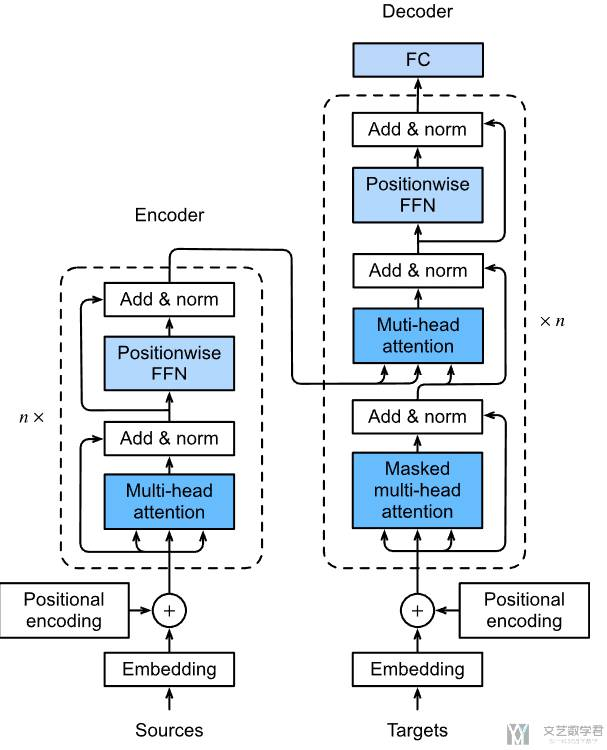## Encoder 部分解释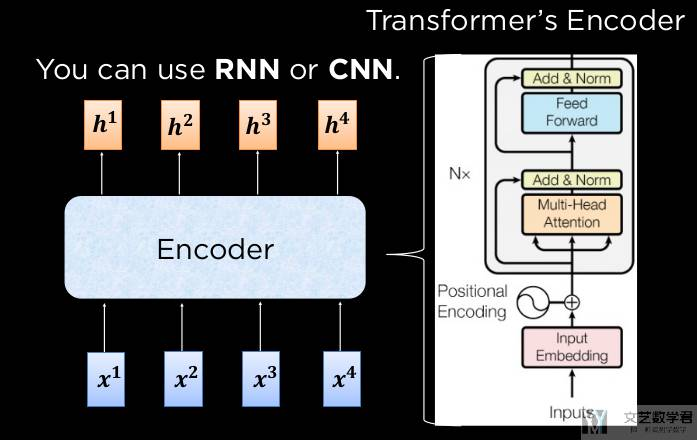### Positionwise Feed-Forward Networks

1. class PositionWiseFFN(nn.Module):
2.     """Positionwise feed-forward network.
3.     输入是三维, 只对最后一维度作处理.
4.     """
5.     def __init__(self, ffn_num_hiddens, ffn_num_outputs):
6.         super().__init__()
7.         self.dense1 = nn.LazyLinear(ffn_num_hiddens)
8.         self.relu = nn.ReLU()
9.         self.dense2 = nn.LazyLinear(ffn_num_outputs)
10.     def forward(self, X):
11.         return self.dense2(self.relu(self.dense1(X)))

1. ffn = PositionWiseFFN(4, 8) # 将 feature dimension 转换为 8
2. ffn.eval()
3. input_x = torch.ones((2, 3, 4))
4. output_x = ffn(input_x)
5. print(f'Input Shape, {input_x.shape}; \nOutput Shape, {output_x.shape};')
6. """
7. Input Shape, torch.Size([2, 3, 4]);
8. Output Shape, torch.Size([2, 3, 8]);
9. """

### Batch Norm 和 Layer Norm 介绍

• `Batch Norm` 是将每个特征（一列），变为均值为 0，方差为 1；
• `Layer Norm` 是将每个样本，变为均值为 0，方差为 1。相当于是对特征进行标准化；

1. ln = nn.LayerNorm(2)
2. bn = nn.LazyBatchNorm1d()
3. X = torch.tensor([[1, 2], [2, 3]], dtype=torch.float32)
4. # Compute mean and variance from X in the training mode
5. print('layer norm:', ln(X), '\nbatch norm:', bn(X))
6. """
7. layer norm: tensor([[-1.0000,  1.0000],
9. batch norm: tensor([[-1.0000, -1.0000],
11. """

2.     """Residual connection followed by layer normalization."""
3.     def __init__(self, norm_shape, dropout):
4.         super().__init__()
5.         self.dropout = nn.Dropout(dropout)
6.         self.ln = nn.LayerNorm(norm_shape)
7.     def forward(self, X, Y):
8.         """_summary_
9.         Args:
10.             X (_type_): 原始的输入
11.             Y (_type_): Y 是 f(X) 的结果, 这里我们加一个 dropout
12.         """
13.         return self.ln(self.dropout(Y) + X)

3. output_x = add_norm(torch.ones((2, 3, 4)), torch.ones((2, 3, 4)))
4. print(f'Output Shape, {output_x.shape}')
5. # Output Shape, torch.Size([2, 3, 4])

### Encoder Block 介绍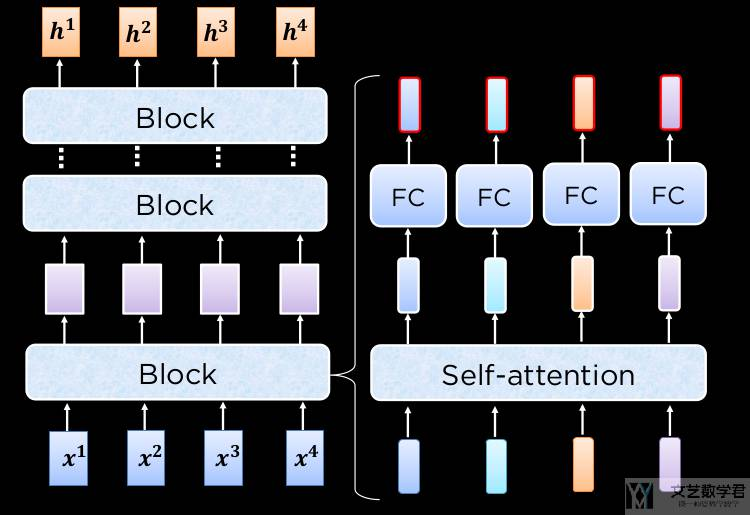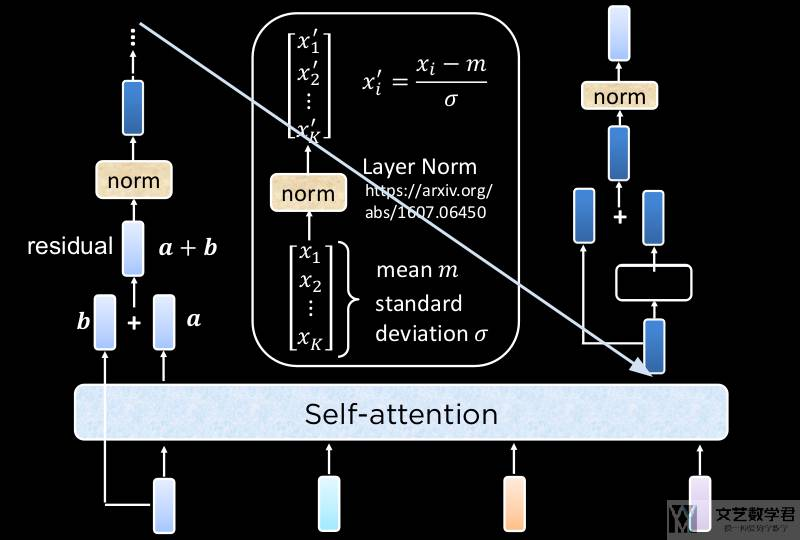1. class TransformerEncoderBlock(nn.Module):
2.     """Transformer encoder block."""
3.     def __init__(self, num_hiddens, ffn_num_hiddens, num_heads, dropout, use_bias=False):
4.         super().__init__()
7.         self.ffn = PositionWiseFFN(ffn_num_hiddens, num_hiddens)
9.     def forward(self, X, valid_lens):
10.         # 计算 self attention 就是 query, key, value 都是 X
11.         self_ttention_result = self.attention(X, X, X, valid_lens) # self attention
12.         Y = self.addnorm1(X, self_ttention_result) # 残差

1. encoder_blk = TransformerEncoderBlock(
2.     num_hiddens=24, ffn_num_hiddens=48,
4. )
5. encoder_blk.eval()
6. X = torch.ones((2, 100, 24))
7. valid_lens = torch.tensor([3, 2])
8. Y = encoder_blk(X, valid_lens)
9. print(f'Output Shape, {Y.shape}')
10. # Output Shape, torch.Size([2, 100, 24])

### Transformer Encoder

1. class TransformerEncoder(Encoder):
2.     """Transformer encoder."""
3.     def __init__(self, vocab_size, num_hiddens, ffn_num_hiddens,
5.         super().__init__()
6.         self.num_hiddens = num_hiddens
7.         self.embedding = nn.Embedding(vocab_size, num_hiddens)
8.         self.pos_encoding = PositionalEncoding(num_hiddens, dropout)
9.         # 加上多个 block 的数据
10.         self.blks = nn.Sequential()
11.         for i in range(num_blks):
13.                 "block"+str(i),
14.                 TransformerEncoderBlock(num_hiddens, ffn_num_hiddens, num_heads, dropout, use_bias)
15.             )
16.     def forward(self, X, valid_lens):
17.         # Since positional encoding values are between -1 and 1,
18.         # the embedding values are multiplied by the square root of the embedding dimension to rescale before they are summed up
19.         # 使得 embedding 的结果的值和 position embedding 的值差不多大
20.         X = self.pos_encoding(self.embedding(X) * math.sqrt(self.num_hiddens))
21.         self.attention_weights = [None] * len(self.blks)
22.         for i, blk in enumerate(self.blks):
23.             X = blk(X, valid_lens)
24.             self.attention_weights[i] = blk.attention.attention.attention_weights
25.         return X

1. encoder = TransformerEncoder(200, 24, 48, 8, 2, 0.5)
2. X = torch.ones((2, 100), dtype=torch.long)
3. valid_lens = torch.tensor([3, 2])
4. Y = encoder(X, valid_lens)
5. print(f'Output Shape, {Y.shape}') # 最后每个字多一个 24 维度的输出
6. # Output Shape, torch.Size([2, 100, 24])

## Decoder 部分解释

`Decoder` 部分，我们不能像在 `Encoder` 的时候一样，看到全部的输入，因此我们每次计算 `attention` 的时候，需要遮住一部分。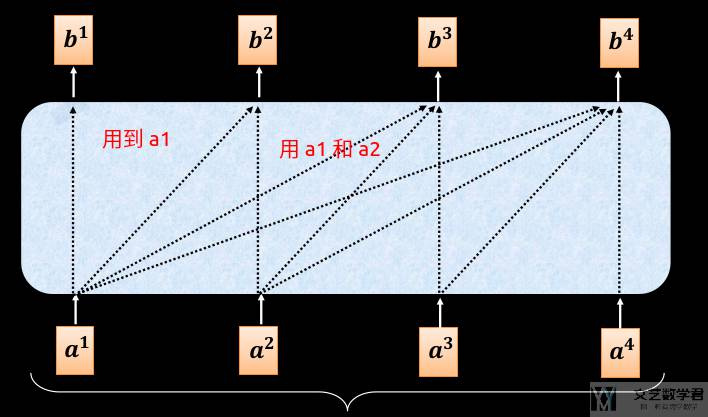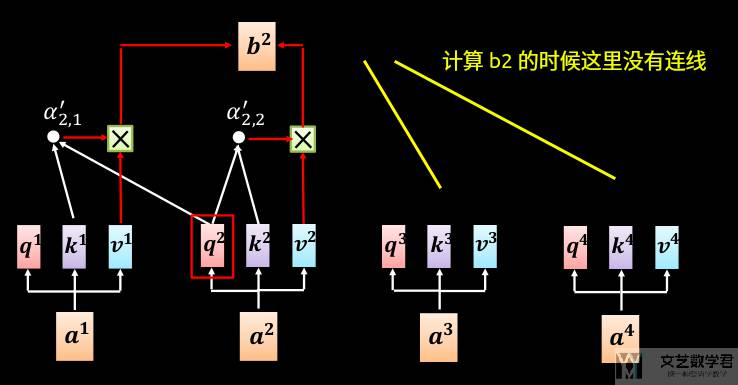### Cross Attention

`Deocder Block` 中，包含两个 `Multi-head Attention`，其中第一个是 `Self-Attention`，这个与前面介绍的一样。第二个是 `Cross Attention`，他的 `key-value` 是来自 `Encoder` 的。`Cross Attention`如下所是：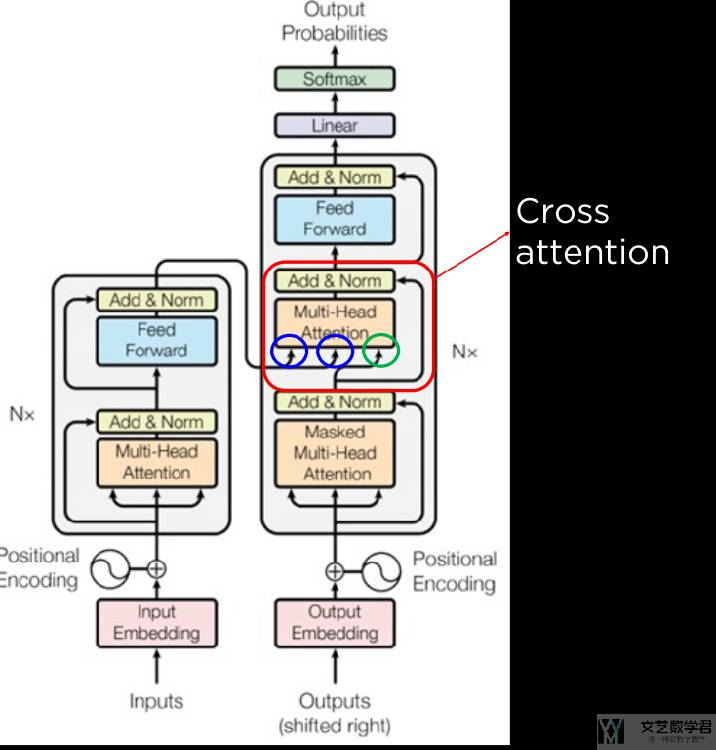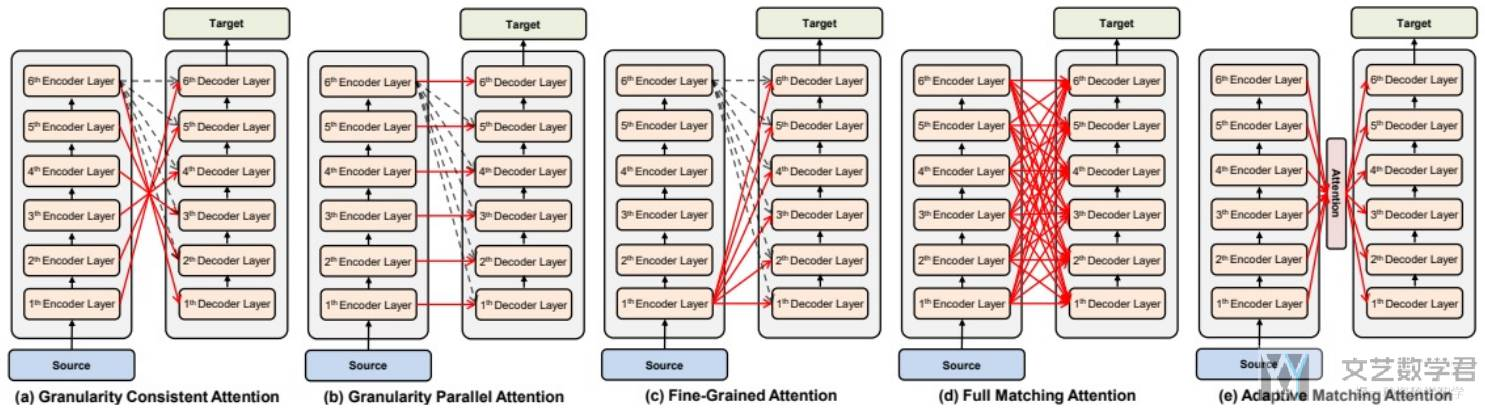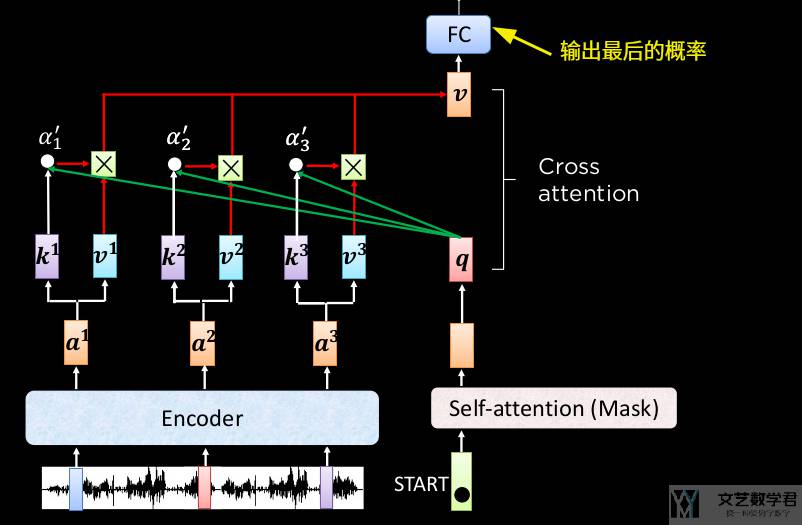• 微信公众号
• 关注微信公众号
•• QQ群
• 我们的QQ群号
•• 本文由 发表于 2022年10月24日07:58:14
• 转载请务必保留本文链接：https://mathpretty.com/15528.html